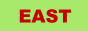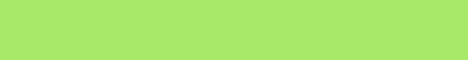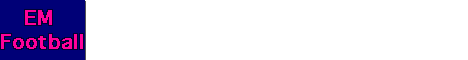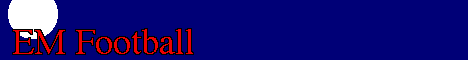### To enter a link to this site, please "copy and paste" the following html code.For the above graphic use the following HTML:For the above graphic use the following HTML:For the above graphic use the following HTML:For the above graphic use the following HTML:For the above graphic use the following HTML:For the above graphic use the following HTML:For the above graphic use the following HTML: